## Tamilnadu Samacheer Kalvi 11th Maths Solutions Chapter 10 Differentiability and Methods of Differentiation Ex 10.5

Choose the correct or the most suitable answer from the given four alternative
Question 1.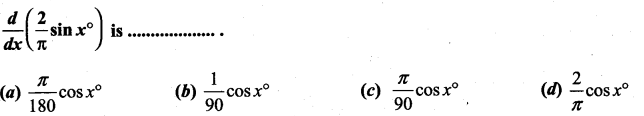Solution:
(b)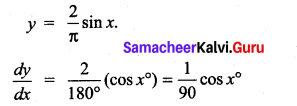Question 2.
If y = f(x2 + 2) and f'(3) = 5, then $$\frac{d y}{d x}$$ at x = 1 is …………….
(a) 5
(b) 25
(c) 15
(d) 10
Solution:
(d)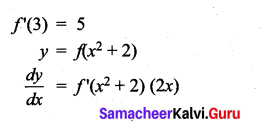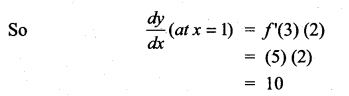Question 3.
If y = $$\frac{1}{4}$$u4, u = $$\frac{2}{3}$$x3 + 5, then $$\frac{d y}{d x}$$ is ……………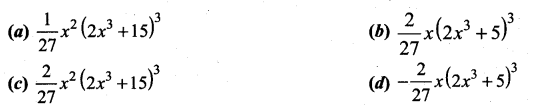Solution:
(c)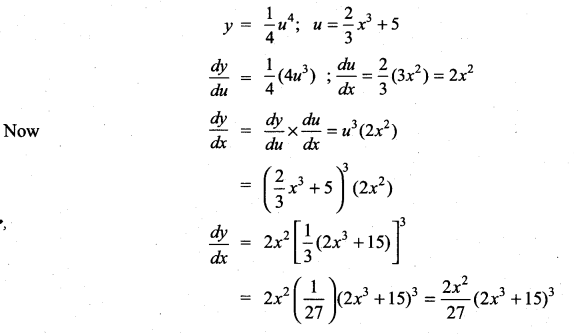Question 4.
If f(x) = x2 – 3x, then the points at which f(x) = f'(x) are …………………..
(a) both positive integers
(b) both negative integers
(c) both irrational
(d) one rational and another irrational
Solution:
(c)
f(x) = x2 – 3x
f'(x) = 2x – 3
Given f(x) = f'(x)
⇒ x2 – 3x = 2x – 3
⇒ x2 – 5x + 3 = 0
x = $$\frac{5 \pm \sqrt{25-12}}{2}=\frac{5 \pm \sqrt{13}}{2}$$
⇒ The roots are irrational

Question 5.
If y = $$\frac{1}{a-z}$$, then $$\frac{d z}{d y}$$ is ……………….
(a) (a – z)2
(b) -(z – a)2
(c) (z + a)2
(d) -(z + a)2
Solution:
(a)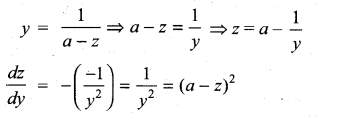Question 6.
If y = cos (sin x2), then $$\frac{d y}{d x}$$ at x = $$\sqrt{\frac{\pi}{2}}$$ is …………..
(a) -2
(b) 2
(c) -2$$\sqrt{\frac{\pi}{2}}$$
(d) 0
Solution:
(d)
y = cos (sin x2)
$$\frac{d y}{d x}$$ = – sin (sin x2) [cos (x2)] (2x)
∴ $$\frac{d y}{d x}$$ at x = $$\sqrt{\frac{\pi}{2}}$$ = -sin (1)  = 0

Question 7.
If y = mx + c and f(0) = f'(0) = 1, then f(2) is ………………
(a) 1
(b) 2
(c) 3
(d) -3
Solution:
(c)
y = mx+c
$$\frac{d y}{d x}$$ = m
y = x + c (i.e.) f(x) = x + c
y(a tx = 0) = f(0) 0 + c = 1 ⇒ c = 1
y = x + 1 ⇒ f(x) = x + 1
f(2) = 2 + 1 = 3

Question 8.
If f(x) = x tan-1x, then f'(1) is ……………
(a) 1 + $$\sqrt{\frac{\pi}{4}}$$
(b) $$\frac{1}{2}+\frac{\pi}{4}$$
(c) $$\frac{1}{2}-\frac{\pi}{4}$$
(d) 2
Solution:
(b)
f(x) = x tan-1 x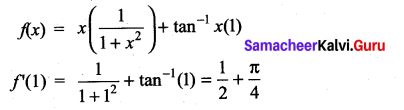Question 9.
$$\frac{d}{d x}$$(ex+5logx) is ……………..
(a) ex.x4 (x + 5)
(b) ex.x (x + 5)
(c) ex + $$\frac{5}{x}$$
(d) ex – $$\frac{5}{x}$$
Solution:
(a)
y = ex+5logx = ex.e5logx = ex.elogx5
= x5 ex
∴ $$\frac{d y}{d x}$$ = x5 (ex) + ex (5x4)
= ex. x4 (x + 5)

Question 10.
If the derivative of (ax – 5) e3x at x = 0 is -13, then the value of a is …………….
(a) 8
(b) -2
(c) 5
(d) 2
Solution:
(d)
y = (ax – 5)e3x
$$\frac{d y}{d x}$$ = y’ = (ax – 5) (3e3x) + e3x (a)
= e3x[3ax – 15 + a]
Given $$\frac{d y}{d x}$$ = -13 at x = 0
⇒ [-15 + a] = -13
⇒ a = -13 + 15
a = 2

Question 11.
x = $$\frac{1-t^{2}}{1+t^{2}}$$, y = $$\frac{2 t}{1+t^{2}}$$ then $$\frac{d y}{d x}$$ is …………..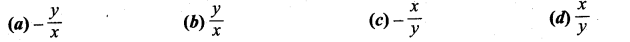Solution:
(c)
Given x = $$\frac{1-t^{2}}{1+t^{2}}$$ and y = $$\frac{2 t}{1+t^{2}}$$
when we put t = tan θ
Then x = cos 2θ and y = sin 2θ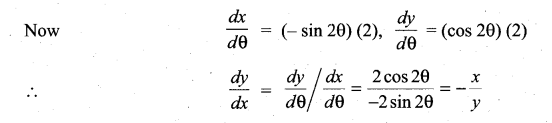Question 12.
If x = a sin θ and y = b cos θ, then $$\frac{d^{2} y}{d x^{2}}$$ is …………..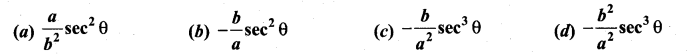Solution:
(c)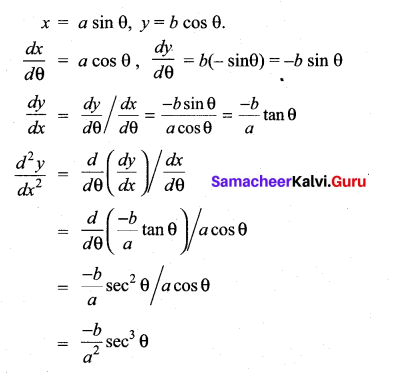Question 13.
The differential coefficient of log10x with respect to logx 10 is …………….
(a) 1
(b) -(log10x)2
(c) (logx 10)2
(d) $$\frac{x^{2}}{100}$$
Solution:
(b)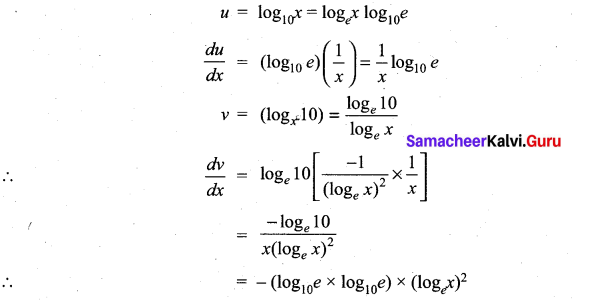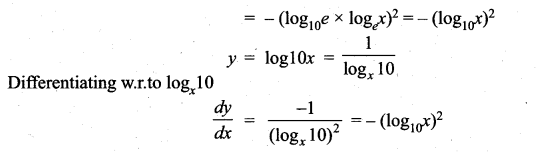Question 14.
If f(x) = x + 2, then f'(f(x)) at x = 4 is ……………..
(a) 8
(b) 1
(c) 4
(d) 5
Solution:
(b)
f(x) = x + 2
f'(x) = 1
f'(x) (at x = 4) = 1Question 15.
If y = $$\frac{(1-x)^{2}}{x^{2}}$$, then $$\frac{d y}{d x}$$ is ………………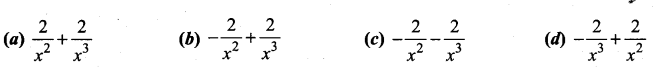Solution:
(d)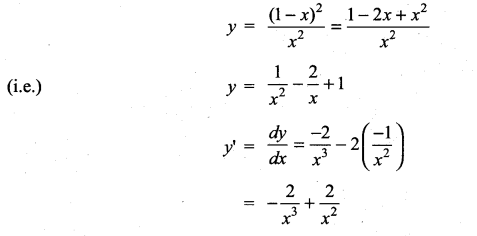Question 16.
If pv = 81, then $$\frac{d p}{d v}$$ at v = 9 is ………….
(a) 1
(b) -1
(c) 2
(d) -2
Solution:
(b)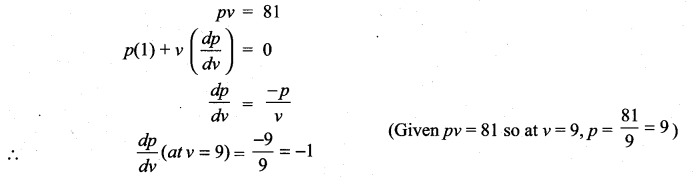Question 17.
If f(x) =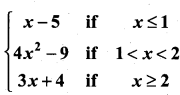then the right hand derivative of f(x) at x = 2 is ……………….
(a) 0
(b) 2
(c) 3
(d) 4
Solution:
(c)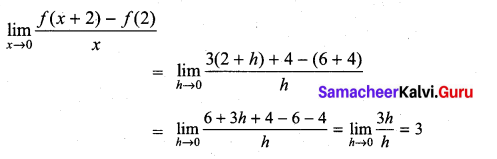Question 18.
It is given that f'(a) exists, then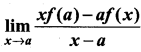is ……………..
(a) f(a) – af'(a)
(b) f ‘(a)
(c) -f ‘(a)
(d) f(a) + af ‘(a)
Solution:
(a)

Question 19.
If f(x) =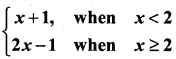then f ‘(2) is ………………
(a) 0
(b) 1
(c) 2
(d) does not exist
Solution:
(d)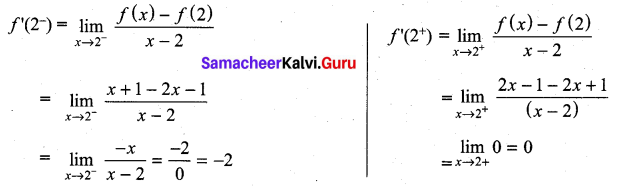∴ f ‘(2) does not existQuestion 20.
If g(x) = (x2 + 2x + 3) f(x) and f(0) = 5 and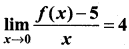then g ‘(θ) is ……………
(a) 20
(b) 22
(c) 18
(d) 12
Solution:
(b) 22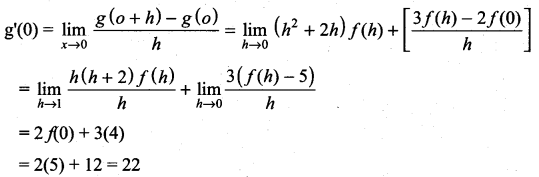Question 21.
If f(x) =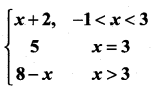, then at x = 3, f ‘(x) is ………………
(a) 1
(b) -1
(c) 0
(d) does not exist
Solution:
(d)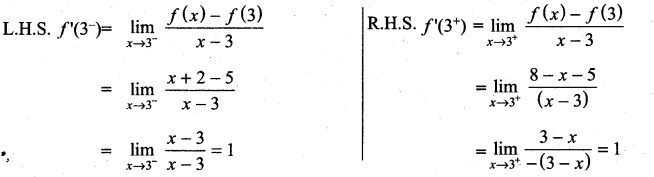as LHS ≠ RHS limit does not exist

Question 22.
The derivative of f(x) = x|x| at x = -3 is …………..
(a) 6
(b) -6
(c) does not exist
(d) 0
Solution:
(a)
f(x) = x|x|
f(x) = x(-x) ⇒ f(x) = – x2
f ‘(x) = -(2x)
f ‘(-3) = -(2) (-3) = 6Question 23.
If f(x) =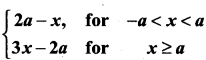, then which one of the following is true?
(a) f(x) is not differentiable at x = a
(b) f(x) is discontinuous at x = a
(c) f(x) is continuous for all x in R
(d) f(x) is differentiable for all x ≥ a
Solution:
(a)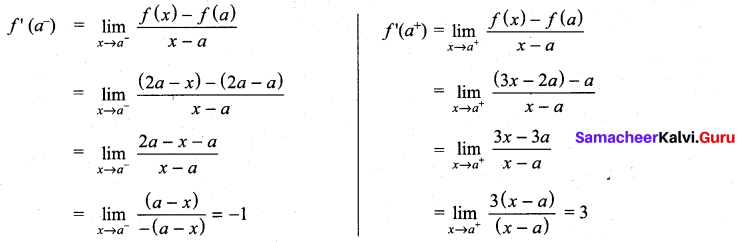f(x) is not differentiable at x = a

Question 24.
If f(x) =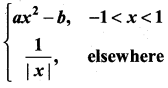is differentiable at x = 1, then ………………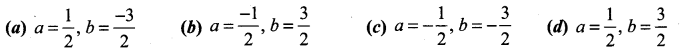Solution:
(c)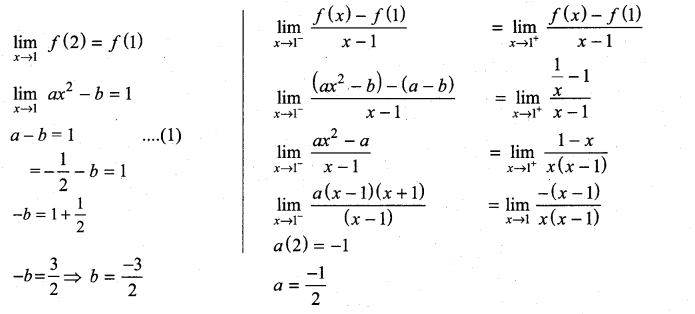Question 25.
Then number of points in R in which the function f(x) = |x – 1| + |x – 3| + sin x is not differentiable, is ……………..
(a) 3
(b) 2
(c) 1
(d) 4
Solution:
(b) 2
f(x) = |x – 1| + |x – 3| + sin x is not differentiable at x = 1, and x = 3